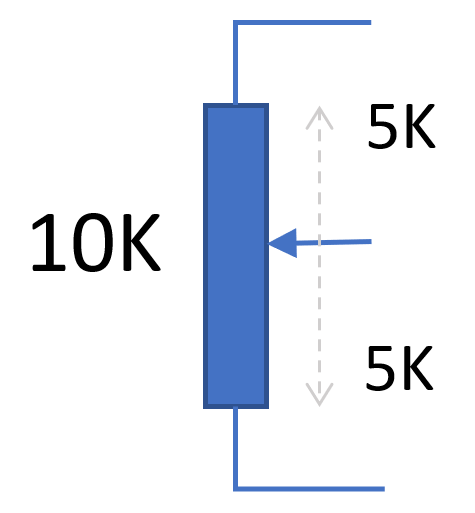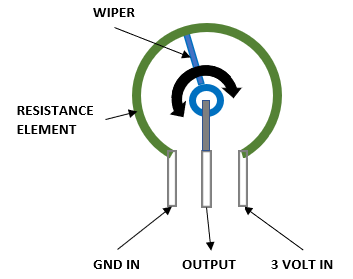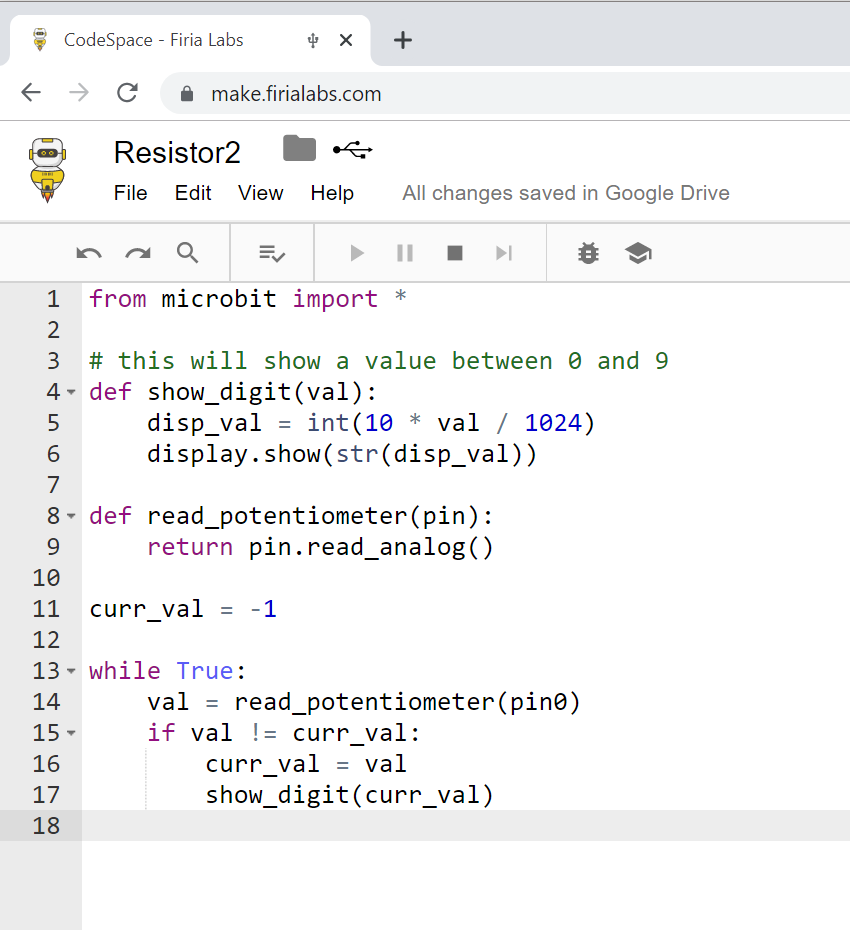×

We will use this information to send you occasional updates.
Firia Labs will never share your information with third parties.

# Potentiometers with Python - Analog Control Peripheral with Potential!## Potentiometers

This fifth installment of our micro:bit peripherals series is an overview of potentiometers.  Potentiometers have a wide range of uses. They can be used to change the volume on a radio, sense the position of a joystick, or change the amount of power an electric car is supplying when you step on the accelerator pedal. There are also many different types:  there are rotary versions and sliders, linear and logarithmic output types. We are going to focus on the rotary, 3-terminal connection, single track, variable resistance potentiometer for this post, but many of the same concepts will apply to any type of potentiometer.  This particular type is the most common – for example it’s what you’d typically find controlling the volume on a car’s radio.

Check out the video demo here: https://youtu.be/GjSfesSpjXs

READING AN ANALOG SIGNAL ON A MICRO:BIT:

In our article on buttons and switches we learned how to read a digital input.  A digital input is either high or low.  It is used to answer the question: Is the switch ON or OFF?  But what if I want to read a value that isn’t just high or low?  What if I want to know where a potentiometer is currently set?  For that we use the function:

``pin0.read_analog()``

This function gives us a value between 0 and 1023. (See the binary help topic in CodeSpace for details on why 1024 is a magic number of levels!) The value will be 0 if the voltage read on the pin is near 0 Volts (GND).  The value will be 1023 if the voltage read on the pin is near the micro:bit’s power supply voltage (ex: 3 Volt battery pack).  Any voltage in between GND and 3 Volts will return a value proportionally between 0 and 1023.  For example if the analog voltage is half of the power supply voltage, you get the integer value:

Value = (1.5 / 3.0) * 1023  511

So, how do you use a potentiometer to produce a voltage between 0 and 3 Volts?

VOLTAGE DIVIDER

Another name for Voltage is “electric potential”, which relates to the familiar physics concept of potential energy. The potentiometer is named for its ability to vary the electric potential by acting as a voltage divider. Take a look at the schematic symbol for a 10KΩ potentiometer below and you can see how this works.

A potentiometer is basically a resistor with a moving tap in the middle, forming two resistors in series. When it’s in the center position, those two resistances are equal – in the diagram below that’s 5K each. So the voltage at the center terminal would be half of the voltage across the outer two.Now look what happens if you move the tap closer to one end. The total resistance remains 10K, so the voltage divider is 1/10 for top-to-center and 9/10 for the center-to-bottom voltages.HOW A ROTARY POTENTIOMETER WORKS:

A standard rotary potentiometer is nothing more than a variable resistor that changes as you turn the knob. For a more in depth understanding of the internals of a rotary potentiometer, take a look at the following diagram:As the potentiometer’s knob spins it turns a small wiper clockwise or counterclockwise. The wiper’s position on the resistance element controls the proportion of total resistance seen between the center and outer two terminals. As the potentiometer’s knob is turned clockwise in the above diagram, the OUTPUT voltage increases since there’s less resistance between it and 3 Volts. When it’s all the way clockwise, the OUTPUT terminal is essentially connected to 3 Volts!

CONNECTING THE DEVICE:

First, to use the potentiometer you must connect its three pins to the micro:bit.  One of the outer potentiometer pins will be connected to the micro:bit’s GND pin, the center potentiometer pin must be connected to a micro:bit input pin, and the last potentiometer pin will be connected to the micro:bit’s 3V pin.HOW TO RUN THIS EXAMPLE:

When running any of the code samples below you should:

1. Connect the Potentiometer as shown in the CONNECTING THE DEVICE section above.
2. Type one of the code examples below into https://make.firialabs.com
3. Run the example - turning the potentiometer will change the display on the micro:bit

If you haven't tried CodeSpace with the micro:bit, you're missing out on a FANTASTIC coding experience! Give it a try!

CODE EXAMPLE 1: BARE MINIMUM CODE

Just scroll the raw ADC value on the micro:bit's LED display. This is a great place to start with any analog sensor. You should see the value go up to 1023 and down to zero. It's possible your potentiometer won't make it quite all the way to these limits due to internal resistance, but it should get close.CODE EXAMPLE 2: AN EXAMPLE WITH FUNCTIONSCODE EXAMPLE 3: AN OBJECT ORIENTED “CLASS” EXAMPLE